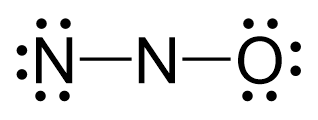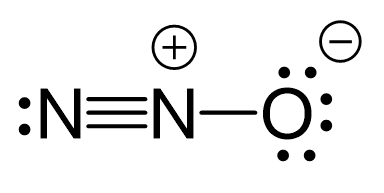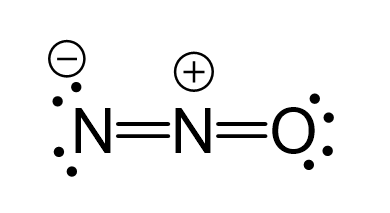## Examples

Oxygen is more electronegative, therefore, it goes in a terminal position:There are 2×5 + 6 = 16 valence electrons and four are already taken to make two bonds. The remaining 12 are distributed between the oxygen and nitrogen:Because the middle nitrogen lacks an octet, two lone pairs from the nitrogen are used to make two pi bonds with the middle nitrogen and the Lewis structure of this compound can be given as follows:There are formal charges here because the middle nitrogen and the oxygen are not in their common bonding pattern:This is one resonance structure and the other would be:There are formal charges here because the middle nitrogen and the oxygen are not in their common bonding pattern:This is one resonance structure and the other would be:In both cases, the central atom has two atoms and no lone pairs, therefore, both geometries are linear.

Steric number 2 corresponds to sp-hybridization where the bond angles are 180o.